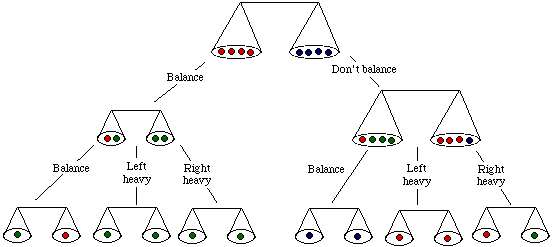Quandaries and Queries

My Grandson (age 15 in the 9th grade) has asked me the following questions and I told him I would try to find out how to solve them.
1. There are 12 coins which look exactly the same, however one of them is heavier than the rest. Using a pointer balance scale, can you identify the odd coin in 3 weighings?
2. Using problem 1 above, follow the same directions except the odd coin could be lighter or heavier.
Your help would be appreciated.

Hi Jack

I will give you a detailed answer to question 2. This also answers question 1, but question 1 can be done in a number of ways. Can you do it starting with 5 coins on each side? 6 on each side?First weighing
Weigh 4 against 4, leaving the remaining 4 aside. Mark the coins on the left red, those on the right blue and the coins left aside green.
The first weighing balances.
In this case we know that the odd coin is green.

### Second weighing

Weigh one red and one green against two green.
• If they balance then the odd coin is the one green that has not been weighed.

#### Third weighing

Weigh the unweighed green against any other to see if it is light or heavy.

• If, on the second weighing, the left side is heavy then either the green coin on the left is too heavy or one of the green coins on the right is too light.

#### Third weighing

Weigh the two green coins that were in the right pan on the second weighing. If they balance then the green coin in the left pan on the second balance is the odd coin and it is heavy. If they don't balance then the lighter of the two is the odd coin.

• If, on the second weighing, the left side is light then either the green coin on the left is too light or one of the green coins on the right is too heavy.

#### Third weighing

Weigh the two green coins that were in the right pan on the second weighing. If they balance then the green coin in the left pan on the second balance is the odd coin and it is light. If they don't balance then the heavier of the two is the odd coin.

The first weighing does not balance.
In this case we know that the odd coin is not green.

### Second weighing

Weigh one red and three green against three red and one blue.
• If they balance then one of the three blues not weighed on the second weighing is the odd coin, and you can tell by the first weighing whether it is too light or too heavy.

#### Third weighing

Weigh two of these blue coins. If they balance the third is the odd one and if they don't balance you know which is the odd coin.

• If the left side is heavy then either the red coin on the left is heavy or the blue coin on the right is light.

#### Third weighing

Weigh the red coin on the left side in the second weighing against any other red coin. If they balance then the blue coin on the right in the second weighing is the odd coin and it is light. If the two reds don't balance then the heavier is the odd coin.

• If the right side is heavy then either the red coin on the left is light or the blue coin on the right is heavy.

#### Third weighing

Weigh the red coin on the left side in the second weighing against any other red coin. If they balance then the blue coin on the right in the second weighing is the odd coin and it is heavy. If the two reds don't balance then the lighter is the odd coin.

Cheers
Harley

Go to Math Central